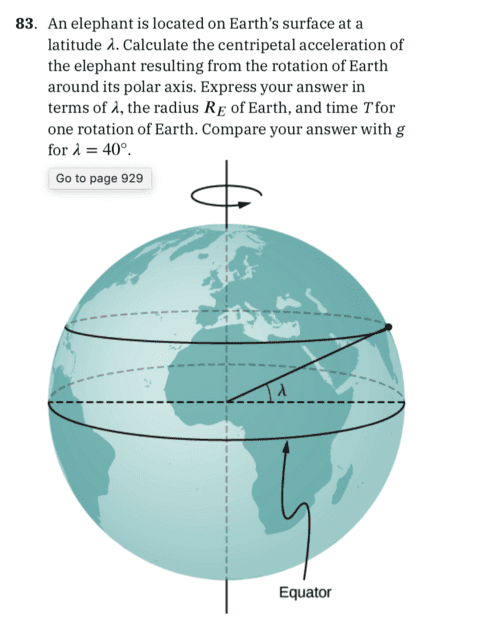# Centripetal acceleration along a latitude of Earth

• mncyapntsi
In summary, the conversation discusses a problem with an equation that is slightly different from the provided textbook solution. The individual is having trouble finding an algebra mistake and asks for help. The conversation also suggests using LaTex for clearer equations.

#### mncyapntsi

Homework Statement
An elephant is located on Earth’s surface at a latitude lambda Calculate the centripetal acceleration of the elephant resulting from the rotation of Earth around its polar axis. Express your answer in terms of lambda, the radius RE of Earth, and time T for one rotation of Earth. Compare your answer with g for lambda = 40º.
Relevant Equations
ac = v^2/rHello,
I am attempting to correctly solve this problem, however I end up with an equation that is slightly different as the one provided in the textbook solution.
For question (a) I get the same thing, just instead of cos, I have cos^2 and I can't figure out where I went wrong. My process was to go from v = d/t where d = REcos(lambda)pi2, and t = T. Then ac=v^2/r=[(REcos^2(lambda)4pi^2] / T^2.
Any help would be much appreciated!
Thanks!

mncyapntsi said:
instead of cos, I have cos^2
maybe you forgot to divide by r?
Chestermiller said: